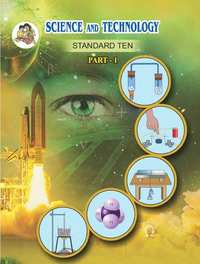# Balbharati solutions for Science and Technology Part 1 10th Standard SSC Maharashtra State Board chapter 1 - Gravitation [Latest edition]

#### Chapters## Chapter 1: Gravitation

[Pages 14 - 15]

### Balbharati solutions for Science and Technology Part 1 10th Standard SSC Maharashtra State Board Chapter 1 Gravitation [Pages 14 - 15]

Q 1 | Page 14

Study the entries in the following table and rewrite them putting the connected items in a single row.

 I II III Mass m/s2 Zero at the centre Weight kg Measure of inertia Acceleration due to gravity Nm2/kg2 Same in the entire universe Gravitational constant N Depends on height
Q 2.1 | Page 14

What is the difference between mass and weight of an object?

Q 2.1 | Page 14

Will the mass and weight of an object on the earth be same as their values on Mars? Why?

Q 2.2 | Page 14

What is the free fall?

Q 2.2 | Page 14

What is the acceleration due to gravity?

Q 2.2 | Page 14

What is escape velocity?

Q 2.2 | Page 14

What is centripetal force?

Q 2.3 | Page 14

Write the three laws given by Kepler. How did they help Newton to arrive at the inverse square law of gravity?

Q 2.4 | Page 14

A stone is thrown vertically upwards with initial velocity u reaches a height ‘h’ before coming down. Show that the time taken to go up is the same as the time taken to come down.

Q 2.5 | Page 14

If the value of g suddenly becomes twice its value, it will become two times more difficult to pull a heavy object along the floor. Why?

Q 3 | Page 15

Explain why the value of g is zero at the centre of the earth.

Q 4 | Page 15

Let the period of revolution of a planet at a distance R from a star be T. Prove that if it was at a distance of 2R from the star, its period of revolution will be $\sqrt{8}$ T.

Q 5.1 | Page 15

An object takes 5 s to reach the ground from a height of 5 m on a planet. What is the value of g on the planet?

Q 5.2 | Page 15

The radius of planet A is half the radius of planet B. If the mass of A is MA, what must be the mass of B so that the value of g on B is half that of its value on A?

Q 5.3 | Page 15

The mass and weight of an object on earth are 5 kg and 49 N respectively. What will be their values on the moon? Assume that the acceleration due to gravity on the moon is 1/6th of that on the earth

Q 5.4 | Page 15

An object thrown vertically upwards reaches a height of 500 m. What was its initial velocity? How long will the object take to come back to the earth? Assume g = 10 m/s

Q 5.5 | Page 15

A ball falls off a table and reaches the ground in 1 s. Assuming g = 10 m/s2, calculate its speed on reaching the ground and the height of the table.

Q 5.6 | Page 15

The masses of the earth and moon are 6 × 1024 kg and 7.4 × 1022 kg, respectively. The distance between them is 3.8 × 105 km. Calculate the gravitational force of attraction between the two? Use G = 6.7 × 10–11 N m2 kg–2

Q 5.7 | Page 15

The mass of the earth is 6 × 1024 kg. The distance between the earth and the sun is 1.5 × 1011 m. If the gravitational force between the two is 3.5 × 1022 N, what is the mass of the sun? Use G = 6.7 × 10–11 N m2 kg–2

## Chapter 1: Gravitation## Balbharati solutions for Science and Technology Part 1 10th Standard SSC Maharashtra State Board chapter 1 - Gravitation

Balbharati solutions for Science and Technology Part 1 10th Standard SSC Maharashtra State Board chapter 1 (Gravitation) include all questions with solution and detail explanation. This will clear students doubts about any question and improve application skills while preparing for board exams. The detailed, step-by-step solutions will help you understand the concepts better and clear your confusions, if any. Shaalaa.com has the Maharashtra State Board Science and Technology Part 1 10th Standard SSC Maharashtra State Board solutions in a manner that help students grasp basic concepts better and faster.

Further, we at Shaalaa.com provide such solutions so that students can prepare for written exams. Balbharati textbook solutions can be a core help for self-study and acts as a perfect self-help guidance for students.

Concepts covered in Science and Technology Part 1 10th Standard SSC Maharashtra State Board chapter 1 Gravitation are Gravitation, Centripetal Acceleration and Centripetal Force, Kepler’s Laws, Newton’s Universal Law of Gravitation, Free Fall, Gravitational Potential Energy, Force, Motion and Rest, Uniform Circular Motion, Acceleration Due to Gravity (Earth’s Gravitational Acceleration), Mass and Weight of an Object.

Using Balbharati 10th Standard Board Exam solutions Gravitation exercise by students are an easy way to prepare for the exams, as they involve solutions arranged chapter-wise also page wise. The questions involved in Balbharati Solutions are important questions that can be asked in the final exam. Maximum students of Maharashtra State Board 10th Standard Board Exam prefer Balbharati Textbook Solutions to score more in exam.

Get the free view of chapter 1 Gravitation 10th Standard Board Exam extra questions for Science and Technology Part 1 10th Standard SSC Maharashtra State Board and can use Shaalaa.com to keep it handy for your exam preparation